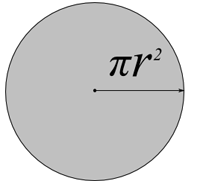#Difference Between Similar Terms and Objects

# Difference Between Area and Surface AreaArea vs Surface Area

Mathematics has ways to make us think, and rethink, and do it all over again. As if mathematics is not confusing enough, brought about by its formulas, operations, and derivations – people can also get confused with definitions, especially with similar terms.

Most of us know geometry is the mathematics of measuring earth, spaces, shape, and figures, and when one thinks of geometry, it is most likely that the term ‘area’ comes to mind.

Area is, commonly, an expression of the size of a 2-dimensional plane. It is expressed in many different units. These units include: square meter, hectare, square kilometer, square foot, square yard, square perch, acre, and square mile, just to name a few.

One of the most basic known formulas of area, is that of a rectangle, which is length multiplied by width (l x w), and in the case of the square, it is length of a side squared (sÂ²).

Other formulas include:

Triangle ‘“ Â½ bh; where b is base and h is height.

Rhombus ‘“ Â½ ab; where a and b are lengths of the two diagonals.

Parallelogram ‘“ bh; where b is the base length, and h is the perpendicular height.

Trapezoid ‘“ Â½ (a + b)h; where a and b are the length of parallel sides, and h is the height.

Circle ‘“ prÂ²; where r is the length of the radius (the square of radius time pi).

Area is often confused with ‘surface area’, which is technically the same if it is in terms of 2-dimensional surfaces. However, it is more appropriately used to express the size of a surface exposed, by a particular solid, that is 3-dimensional. For example, a cube will have a surface area equal to the sum of the areas of all six sides (6sÂ²).

Like area, surface area is also expressed in square units.

Formulas of surface areas of some solids:

Cylinder – 2prÂ²(r + h); where r is radius, and h is the height of the cylinder.

Cone – pr(r + l); where r is the radius, and l is the slant height of the cone.

Sphere ‘“ 4prÂ²; where r is the radius.

Summary:

1. The term area is a general term that expresses the size measurement of a surface, while surface area is more appropriately used to express the measurement of the exposed surface of a particular solid object.

2. Area is for 2-dimensional flat surfaces, while surface area is for 3-dimensional solids.

Latest posts by Ian (see all)

### Search DifferenceBetween.net :

Custom Search

Help us improve. Rate this post!(6 votes, average: 4.33 out of 5)Loading...Email This Post : If you like this article or our site. Please spread the word. Share it with your friends/family.